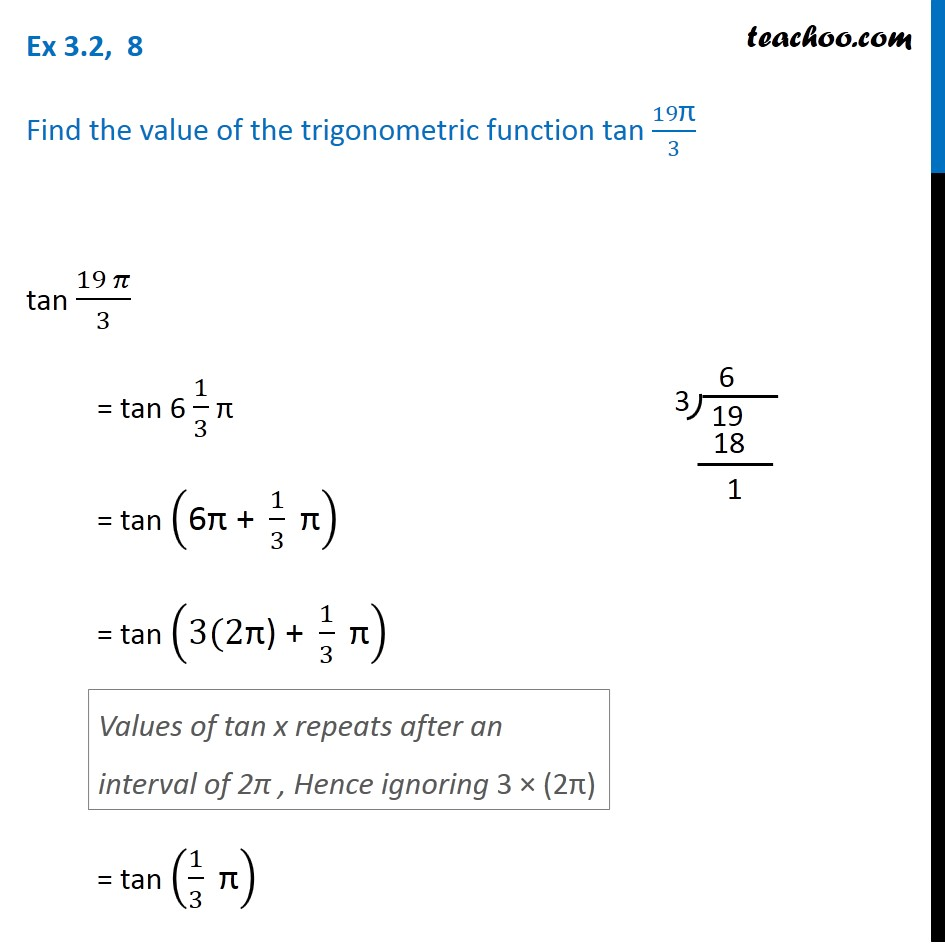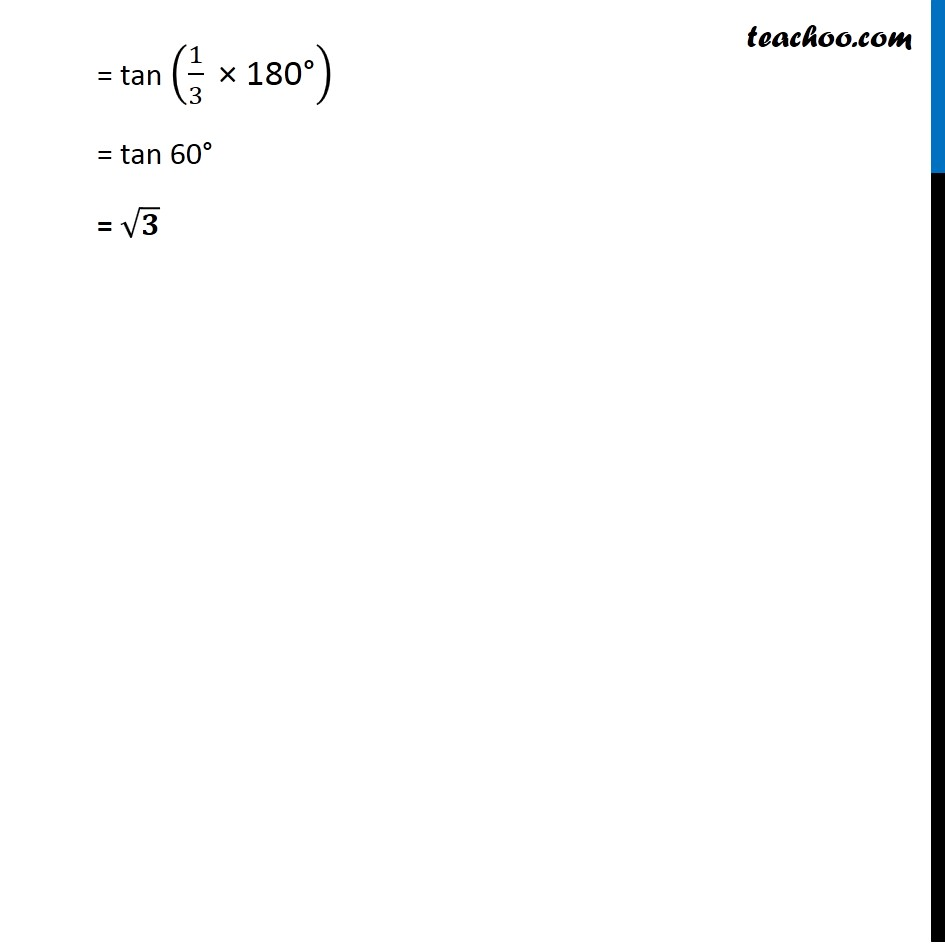1. Chapter 3 Class 11 Trigonometric Functions (Term 2)
2. Serial order wise
3. Ex 3.2

Transcript

Ex 3.2, 8 Find the value of the trigonometric function tan 19"π" /3 tan (19 𝜋)/3 = tan 6 1/3 π = tan ("6π + " 1/3 " π" ) = tan ("3(2π) + " 1/3 " π" ) = tan (1/3 " π" ) Values of tan x repeats after an interval of 2π , Hence ignoring 3 × (2π) = tan (1/3 " × 180°" ) = tan 60"°" = √𝟑